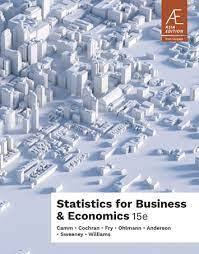•# Statistics for Business and Economics 15E By Jeffrey D. Camm 9789815119329

RM 100.00
- +

1. Data and Statistics.

2. Descriptive Statistics: Tabular and Graphical Displays.

3. Descriptive Statistics: Numerical Measures.

4. Introduction to Probability.

5. Discrete Probability Distributions.

6. Continuous Probability Distributions.

7. Sampling and Sampling Distributions.

8. Interval Estimation.

9. Hypothesis Tests.

10. Inference about Means and Proportions with Two Populations.

12. Comparing Multiple Proportions, Test of Independence and Goodness of Fit.

13. Experimental Design and Analysis of Variance.

14. Simple Linear Regression.

15. Multiple Regression.

16. Regression Analysis: Model Building.

17. Time Series Analysis and Forecasting.

18. Decision Analysis

19. Nonparametric Methods.

20. Statistical Methods for Quality Control.

21. Index Numbers.

22. Sample Survey (online).

Appendix A. References and Bibliography.

Appendix B. Tables.

Appendix C. Summation Notation.

Appendix D. Microsoft Excel and Tools for Statistical Analysis.

Appendix E. Computing p-Values Using JMP and Excel.